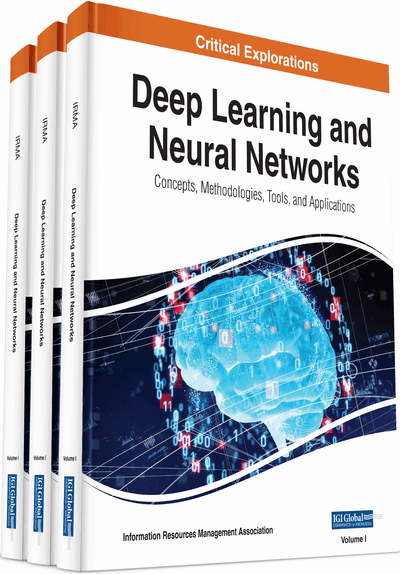# Forecasting of Electricity Demand by Hybrid ANN-PSO Models

Atul Anand, L. Suganthi
DOI: 10.4018/978-1-7998-0414-7.ch048
OnDemand:
(Individual Chapters)
Available
\$37.50
No Current Special Offers

## Abstract

Developing economies need to invest in energy projects. Because the gestation period of the electric projects is high, it is of paramount importance to accurately forecast the energy requirements. In the present paper, the future energy demand of the state of Tamil Nadu in India, is forecasted using an artificial neural network (ANN) optimized by particle swarm optimization (PSO) and by Genetic Algorithm (GA). Hybrid ANN Models have the potential to provide forecasts that perform well compared to the more traditional modelling approaches. The forecasted results obtained using the hybrid ANN-PSO models are compared with those of the ARIMA, hybrid ANN-GA, ANN-BP and linear models. Both PSO and GA have been developed in linear and quadratic forms and the hybrid ANN models have been applied to five-time series. Amongst all the hybrid ANN models, ANN-PSO models are the best fit models in all the time series based on RMSE and MAPE.
Chapter Preview
Top

## Introduction

In order to ensure sustained growth for developing economies such as the state of Tamil Nadu in India, long term planning of energy resources is imperative to bridge the electrical energy gap. The allocation of capital resources requires an accurate model for forecasting electricity demand that can enable the optimal utilization of scarce resources. It is also useful for resource planning and for attracting investments in the field of energy. In recent reports on the energy policies of India, simple measures of GDP-elasticity and energy intensities have been used for demand forecasting for ten or more years (Government of India,2006). In this paper, the total electric energy demand is segregated into agriculture, residential, commercial, and industrial sectors. For each sector, hybrid artificial neural network (hybrid ANN) models have been developed using the state of art optimizing techniques, namely, particle swarm optimization (PSO) and Genetic Algorithm (GA). Hybrid ANN models have the potential to provide forecasts that perform well, compared to the more traditional modeling approaches. For each optimizing technique, both linear and quadratic forms have been developed. The four hybrid ANN models that are compared in this paper are: -ANN-PSO(Linear), ANN-PSO(Quadratic), ANN-GA(Linear) and ANN-GA(Quadratic). The results of each sector are compared with linear, autoregressive integrated moving average (ARIMA), and artificial neural network with backward propagation (ANN-BP) models. The best fit model for each sector is selected based on error indices such as root mean square error (RMSE) and mean absolute percentage error (MAPE).

The study of the electric energy demand profile of the State of Tamil Nadu in Figure 1 shows a non-linear growth with undulations superimposed on the profile. For such a non-linear profile, in the past, many applications used the traditional statistical models, such as the autoregressive model, moving average model and the auto regressive moving average. These models perform well when the data lie within the range of past observations but they perform poorly while predicting extremes and also when the data lies near the limits. Moreover, conventional optimization might either fail to obtain a feasible solution or be trapped in local optima. However, with the advent of ANN and inspired by its strong ability of non-linear mapping, it has been applied to the field of energy forecasting. ANN does not require to specify a particular model form. Rather, the model is adaptively formed based on the features presented from the data. This data driven approach is suited for many empirical data sets where no theoretical guidance is available to suggest an appropriate data generating process. Therefore, ANN has been considered in the present study. Various independent socio-economic factors were considered to develop a forecasting model, as Table 1 shows, and Pearson correlations were found. On this basis, the per capita energy index, number of consumers, and consumer price index were taken as independent variables.

Table 1.
Details of correlations among socio-economic factors
 Pearson Correlations Per Capita Energy Index Consumers Population Consumer Price Index Gross State Domestic Product Per Capita Income Total Electricity Demand 0.68 0.76 0.65 0.8 -0.186 -0.082

## Complete Chapter List

Search this Book:
Reset